# Electronics and Communication Engineering - Power Electronics

Exercise : Power Electronics - Section 9
1.
In a semi converter feeding a resistive load
the waveshapes of output voltage and output current are exactly similar
the waveshapes of output voltage and output current can not be similar
the waveshapes of output voltage and output current are similar only for low values of firing angle
the waveshapes of output voltage and output current may be similar or dissimilar
Explanation:
No answer description is available. Let's discuss.

2.
In an n stage high frequency series inverter having a time period T, each stage is active for a time interval of
T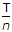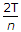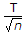Explanation:
No answer description is available. Let's discuss.

3.
In a 3 phase semiconverter, the output voltage becomes discontinuous for
firing angle > 30°
firing angle > 60°
firing angle > 90°
firing angle > 120°
Explanation:
No answer description is available. Let's discuss.

4.
A single phase fully controlled bridge converter is feeding an RLE load. Input voltage is Vm sin ωt and firing angle is a. If average current is I0, then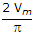cos a = RI0 + E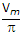cos a = RE0 + Ecos a = E0 - RI0cos a = E - RI0